# A Contribution to Analytical Solutions for Buckling Analysis of Axially Compressed Rectangular Stiffened Panels

A Contribution to Analytical Solutions for Buckling Analysis of Axially Compressed Rectangular Stiffened Panels

Victor Tochukwu IbeabuchiMathias Owus Ibearugbulem Kelechi Okechukwu Njoku Ezekiel Onyinyechi Ihemegbulem Princewill Obumneke Okorie

Department of Civil Engineering, Alex Ekwueme Federal University Ndufu Alike, P.M.B. 1010, Abakaliki, Nigeria

Department of Civil Engineering, Federal University of Technology, P.M.B. 1526, Owerri, Nigeria

Corresponding Author Email:
ibeabuchivictor@gmail.com
Page:
301-306
|
DOI:
https://doi.org/10.18280/rcma.310506
10 May 2021
|
Accepted:
11 August 2021
|
Published:
31 October 2021
| Citation

OPEN ACCESS

Abstract:

Analytical solution to the buckling problems of stiffened panels subjected to in-plane compressive loads is presented. The total potential energy functional of stiffened panel is obtained by the summation of that of a line continuum and stiffened panel derived from elastic principles of mechanics. Minimizing the resulting equation with respect to deflection coefficient and rearranging gives the expression for obtaining the buckling load of stiffened panel. Exact deflection functions were substituted directly in the new solution and various edge conditions were considered in this analysis. Obtained results were compared with analytical results of previous works. The method is computationally efficient for complex edge conditions and gives high numerical accuracy.

Keywords:

analytical approach, buckling, Ritz method, stiffened panels, exact deflection function

1. Introduction

Stiffened panels are widely used in many engineering structures due to enhancement in strength and reduction in weight of the overall structure. As a result, stiffened panels are important structural elements for analysis and design of thin-walled structures. Often times, these thin structural elements are prone to buckling due to the action of high compressive forces. Their buckling and dynamic behaviour has attracted great attention from numerous researchers as in [1, 2].

Relevant codes have given specifications for buckling analysis and design of stiffened panels based on the available literature. Galéa and Martin  presented buckling of stiffened panels in accordance to Eurocode 3 Part 1.5 and developed a general calculation method for critical buckling stress with the help of EBPlate software. Various approaches have been used recently to solve the elastic buckling problems of stiffened panels [4-13]. In the numerical approach, FEM is widely used for buckling analysis problem irrespective of the boundary conditions and loading type. However, convergence of the result depends on the number of finite units created which is time consuming and requires details. In addition, during the preliminary design stage it is not efficient to use FEM, as the dimensions are not in their final configuration [10, 11, 13]. Consequently, some researchers adopted analytical approach in their work.

The two main analytical approaches generally employed for solving buckling behaviour of structures are based on equilibrium theory and the energy theory. Equilibrium approach uses governing equation of equilibrium of forces acting on the plate as its characteristic equation. On the other hand, the characteristic equation for energy approach is the total potential energy functional. Governing equation of equilibrium of forces acting on the plate can be regarded as the resultant force acting on the plate whereas the total potential energy functional is the algebraic sum of the internal (strain) energy of the plate and the external work on the plate.

Exact displacement function is used in equilibrium approach whereas both exact and approximate displacement functions can be used in energy approach. Using exact displacement function in energy approach gives excellent result whereas using approximate displacement function in energy approach gives approximate result. The better the approximate displacement function used in energy approach the better the result. However, approximate displacement function cannot be used in equilibrium approach as the result will be far from exact solution.

Exact displacement function can only be obtained by direct integration of the governing equation. Sometimes displacement functions are assumed and in some cases, exact functions are assumed. When the assumed function is exact, it can be used either in equilibrium approach or in energy approach to get excellent results. However, if the assumed displacement function does not coincide with exact function, applying it in equilibrium approach will give poor result. Applying it in energy approach will give a better result. Governing equation can be obtained by extremizing total potential energy functional with respect to displacement function. The integrand of the extremized functional is the governing equilibrium.

The analytical approach effectively gives exact solutions for buckling problems of stiffened panels. However, despite the fact that several theoretical and experimental studies have been conducted in the past decades to obtain the critical buckling loads of stiffened panels in both uniaxial and biaxial range, some aspects of theories of buckling are still unaddressed largely because of the assumed shape functions and the variation in the number of finite series employed in the analysis [12, 14, 15].

Many previous studies on stiffened panel buckling focused on using Fourier series or trigonometric series to derive the deflection function irrespective of the analytical approach or semi-analytical method used. However, the use of trigonometric series in formulating deflection functions of complex boundary conditions may be very rigorous except for a rectangular stiffened plate with four simply supported edges and in such situations, a simplified approach that will enable a direct substitution of trigonometric shape function may be used in the problem formulation.

This research work presents Modified Ritz Analytical Approach (MRAA) for buckling of rectangular stiffened panels under uniaxial compressive loads. One of the advantages of the present method is the ease in computation using the formulas for stiffness coefficients. Another advantage is that good results are obtained even though the method is of energy approach. More so, since plates would naturally be considered to have failed in the first mode, this method presents a very simple and easy approach that gives excellent results.

The trigonometric functions were substituted to obtain solutions for stiffened panels with all edges simply supported (SSSS), all edges clamped (CCCC) and stiffened panel with two opposite edges clamped and the other opposite edges simply supported (CSCS). Thus, the study is further extended in this investigation to take into account the effects of number of stiffeners. The present analytical results were validated by comparing them with the exact solutions as well as Analytical solutions from previous works. Ritz method is based on variation principles of mechanics and can be used as an approximate method for solutions of mechanics problems .

Wang and Aung  presented p-Ritz method for the plastic buckling analysis of thick plates. They adopted Mindlin plate theory in their analysis and their result shows good convergence. Ritz method employs use of approximate deflection functions. By Modified Ritz Analytical Method, one implies use of exact deflection function in Ritz method.

2. Panel Definition and Boundary Condition

A rectangular stiffened plate with edge numbers was shown in Figure 1. There are two conditions considered. They are simply supported (S) and clamped (C) boundary conditions. SSSS represented a plate with four edges simply supported, CCCC represented a plate with 4 edges clamped and CSCS represented a plate with edges 1 and 3 clamped edges and edges 2 and 4 simply supported.

## 1.png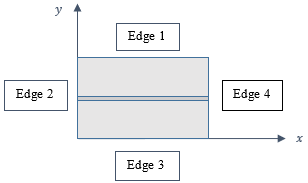Figure 1. Longitudinally rectangular stiffened panel with edge numbers

3. Theoretical Formulations

An isotropic thin rectangular stiffened panel under in-plane uniaxial compressive loads is considered in Figure 2. The compressive loads, Nx act parallel to both x-axis and stiffeners, and three stiffeners divide the panel into four equal parts. In the case of one longitudinal stiffener, the panel is divided into two equal parts.

## 2.png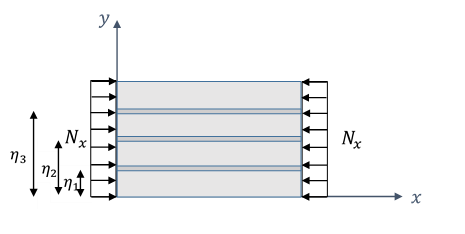Figure 2. Stiffened panel under uniaxial in-plane compression in Cartesian coordinates

An isotropic Variation principle is applied to obtain the buckling solution. Following the work of Ibearugulem et al., , total potential energy functional for a classical rectangular plate is derived as follow:

$\Pi p=\frac{b D}{2 a^{3}} \int_{0}^{1} \int_{0}^{1}\left\{\left(\frac{\partial^{2} w}{\partial R^{2}}\right)^{2}+\frac{2}{P^{2}}\left(\frac{\partial^{2} w}{\partial R \partial Q}\right)^{2}\right.$

$\left.+\frac{2}{P^{2}}\left(\frac{\partial^{2} w}{\partial Q^{2}}\right)^{2}\right\} d R d Q$

$-\frac{b N_{x}}{2 a} \int_{0}^{1} \int_{0}^{1}\left(\frac{\partial w}{\partial R}\right)^{2} d R d Q$             (1)

where: $\Pi_{\mathrm{p}}$ is the total potential for thin rectangular panels, D is the flexural rigidity, w is the deflection function, R and Q are the non dimensional coordinates and P is the aspect ratio expressed as the ratio of length, a to width, b of the panel written as;

$P=a / b$      (2)

Similarly, total potential energy functional for stiffener elements is given by  as:

\begin{aligned} \Pi_{\mathrm{s}}=\frac{E I_{i}}{2 a^{3}} \int_{0}^{1} \int_{0}^{1}\left(\frac{\partial^{2} w}{\partial R^{2}}\right)_{Q=\eta i}^{2} d R d Q & \\-\frac{P_{s i}}{2 a} \int_{0}^{1} \int_{0}^{1}\left(\frac{\partial w}{\partial R}\right)^{2} d R d Q \end{aligned}               (3)

where: $\Pi_{s}$ is the total potential for the stiffeners, $\eta i$ is the distance of the stiffeners from the edge y = 0. Independent coordinates whose lengths in x and y directions are a and b are expressed in the form of non-dimensional coordinates R and Q as;

$y=b Q ; \quad 0 \leq \mathrm{Q} \leq 1$      (4)

$x=a R \quad 0 \leq R \leq 1$       (5)

where;

$\gamma_{i}=\frac{E I_{i}}{b D}=$  Ratio of bending stiffness rigidity of stiffeners to the plate.

$\delta_{i}=\frac{P_{s i}}{b N_{x}}=\frac{A_{i}}{b h}$  Ratio of cross-sectional area of the stiffeners to the plate.

The total potential energy functional for the stiffened panel is obtained by the summation of Eq. (1) and Eq. (3). Minimizing the resulting equation and making $N_{x}$ the subject gave:

$N_{x(c r i)}=\frac{\frac{D}{(a)^{2}}\left[\mathrm{~K}_{\mathrm{RR}}+2 P^{2} \mathrm{~K}_{\mathrm{RQ}}+\mathrm{P}^{4} \mathrm{~K}_{\mathrm{QQ}}+\sum_{i=1}^{n} \gamma_{i}\left(\mathrm{~K}_{\mathrm{RR}}\right)_{Q=\eta i}\right]}{\left[\mathrm{K}_{\mathrm{R}}+\sum_{i=1}^{n} \delta_{i}\left(\mathrm{~K}_{\mathrm{R}}\right)_{Q=\eta i}\right]}$                   (6)

Stiffness coefficients parameters, KRR, KRQ, KQQ KR are defined by Eqns. (8)-(10).

Eq. (6) can be written in terms of length, b as in Eq. (7)

$N_{x(c r i)}=\frac{\frac{D}{b^{2}}\left[\frac{1}{P^{2}} \cdot \mathrm{K}_{\mathrm{RR}}+2 \mathrm{~K}_{\mathrm{RQ}}+\mathrm{P}^{2} \mathrm{~K}_{\mathrm{QQ}}+\frac{1}{P^{2}} \cdot \sum_{i=1}^{n} \gamma_{i}\left(\mathrm{~K}_{\mathrm{RR}}\right)_{Q=\eta i}\right]}{\left[\mathrm{K}_{\mathrm{R}}+\sum_{i=1}^{n} \delta_{i}\left(\mathrm{~K}_{\mathrm{R}}\right)_{Q=\eta i}\right]}$             (7)

where:

For $w=h_{R} \cdot h_{Q}$

$\mathrm{K}_{\mathrm{RR}}=\int_{0}^{1} \int_{0}^{1}\left(\frac{\partial^{2} h_{R}}{\partial R^{2}} \cdot \mathrm{h}_{\mathrm{Q}}\right)^{2} \partial R \partial Q ;$

$\mathrm{K}_{\mathrm{RQ}}=\int_{0}^{1} \int_{0}^{1}\left(\frac{\partial h_{R}}{\partial \mathrm{R}} \cdot \frac{\partial h_{Q}}{\partial \mathrm{Q}}\right)^{2} \partial R \partial Q$           (8)

\begin{aligned} \mathrm{K}_{\mathrm{QQ}}=\int_{0}^{1} \int_{0}^{1}\left(\frac{\partial^{2} h_{Q}}{\partial Q^{2}} \cdot \mathrm{h}_{\mathrm{R}}\right)^{2} \partial R \partial Q & \\\left(\mathrm{~K}_{\mathrm{RR}}\right)_{Q=\eta i}=\eta \eta \mathrm{i} \int_{0}^{1}\left(\frac{\partial^{2} h_{R}}{\partial R^{2}}\right)^{2} \partial R \end{aligned}               (9)

$\eta \eta \mathrm{i}=\int_{0}^{1}\left(h_{Q \eta i}\right)^{2} \partial Q=\left(h_{Q \eta i}\right)^{2}$ when $\mathrm{Q}=\eta i$         (10)

Thus;

$\eta \eta \mathrm{i} . \mathrm{K}_{\mathrm{rr}}=\left(\mathrm{K}_{\mathrm{RR}}\right)_{Q=\eta i} ; \mathrm{K}_{\mathrm{rr}}=\int_{0}^{1}\left(\frac{\partial^{2} h_{R}}{\partial R^{2}}\right)^{2} \partial R$            (11)

$\mathrm{K}_{\mathrm{R}}=\int_{0}^{1} \int_{0}^{1}\left(\frac{\partial h_{R}}{\partial \mathrm{R}} \cdot \mathrm{h}_{\mathrm{Q}}\right)^{2} \partial R \partial Q$

$\left(\mathrm{~K}_{\mathrm{R}}\right)_{Q=\eta i}=\eta \eta_{0}^{1} \int_{0}^{2}\left(\frac{\partial h_{R}}{\partial \mathrm{R}}\right)^{2} \partial R$         (12)

Thus;

$\eta \eta$ i. $K_{\mathrm{r}}=\left(K_{\mathrm{R}}\right)_{Q=\eta i} ; \mathrm{K}_{\mathrm{r}}=\int_{0}^{1}\left(\frac{\partial h_{R}}{\partial \mathrm{R}}\right)^{2} \partial R$          (13)

Substituting the exact deflection functions into Eqns. (8)-(13) gives the exact stiffness coefficients presented in Table 1.

Table 1. Stiffness coefficients for plates and stiffeners

 Plate Plates Stiffness Coefficients KRR KRQ KQQ KR SSSS ¼(ᴫ4) ¼(ᴫ4) ¼(ᴫ4) ¼(ᴫ2) CSCS ¾ (ᴫ4) ᴫ4 4(ᴫ4) ¾ (ᴫ2) CCCC 12(ᴫ4) 4(ᴫ4) 12(ᴫ4) 3(ᴫ2) Stiffener Stiffeners Stiffness Coefficients Krr Kr S - S ½( ᴫ4) ½( ᴫ2) C - C 8(ᴫ4) 8(ᴫ2)

The exact deflection functions for three plates of different boundary conditions (SSSS, CCCC and CSCS) are given as follows;

SSSS panel: $w=\operatorname{Sin} \pi R . \operatorname{Sin} \pi Q ; h_{R}=\operatorname{Sin} \pi R ; h_{Q}$

$=\operatorname{Sin} \pi Q$

CCCC panel: $w=(1-\operatorname{Cos} 2 \pi R) \cdot(1-\operatorname{Cos} 2 \pi Q) ; h_{R}$

$\quad=1-\operatorname{Cos} 2 \pi R ; h_{Q}=1-\operatorname{Cos} 2 \pi Q$

CSCS d panel: $w=\operatorname{Sin} \pi R \cdot(1-\operatorname{Cos} 2 \pi Q) ; h_{R}$

$=\operatorname{Sin} \pi R ; h_{Q}=1-\operatorname{Cos} 2 \pi Q$

Applying values in Table 1 into Eq. (7) with respect to boundary conditions gave buckling solutions for stiffened panels under in plane compressive load as given below;

3.1 Stiffened SSSS plates

For one stiffener

$\eta i=\eta 1=0.5$ and $h_{Q=\eta 1}=\sin \pi \times 0.5=\sin \frac{\pi}{2}=1$       (14)

$\eta \eta \mathrm{i}=\int_{0}^{1}\left(h_{Q \eta i}\right)^{2} \partial Q=\left(h_{Q \eta i}\right)^{2}=1$        (15)

$N_{x(c r i)}=\pi^{2} \frac{D}{b^{2}} \times \frac{\left(1+P^{2}\right)^{2}+2 \gamma}{P^{2}[1+2 \delta]}$        (16)

For two stiffeners

$\eta 1=\frac{1}{3}$ and $h_{Q=\eta 1}=\operatorname{Sin} \pi \times \frac{1}{3}=\operatorname{Sin} \frac{\pi}{3}=0.866025$      (17)

$\eta \eta 1=\int_{0}^{1}\left(h_{Q \eta i}\right)^{2} \partial Q=\left(h_{Q \eta i}\right)^{2}=(0.866025)^{2}=0.75$        (18)

$\eta 2=\frac{2}{3}$ and $h_{Q=\eta 2}=\operatorname{Sin} \pi \times \frac{2}{3}=\operatorname{Sin} \frac{2 \pi}{3}=0.866025$         (19)

$\eta \eta 2=\int_{0}^{1}\left(h_{Q \eta i}\right)^{2} \partial Q=\left(h_{Q \eta i}\right)^{2}=(0.866025)^{2}=0.75$        (20)

$N_{x(c r i)}=\frac{\pi^{2} D}{b^{2}} \times \frac{\left(1+P^{2}\right)^{2}+3 \gamma}{P^{2}[1+3 \delta]}$       (21)

For three stiffeners

Similarly, for three longitudinal stiffeners dividing the stiffened panel into four equal parts;

$N_{x(c r i)}=\frac{\pi^{2} D}{b^{2}} \times \frac{\left(1+P^{2}\right)^{2}+4 \gamma}{P^{2}[1+4 \delta]}$      (22)

3.2 Stiffened CSCS plates

One stiffener

$N_{x(c r i)}=\frac{\pi^{2} D}{b^{2}} \times \frac{\left[1+\frac{8}{3} P^{2}+\frac{16}{3} P^{4}+\frac{8}{3} \gamma\right]}{P^{2}\left[1+\frac{8}{3} \delta\right]}$       (23)

Two stiffeners

$N_{x(\text { cri })}=\frac{\pi^{2} D}{b^{2}} \times \frac{\left[1+\frac{8}{3} P^{2}+\frac{16}{3} \mathrm{P}^{4}+3 \gamma\right]}{P^{2}\lceil 1+3 \delta\rceil}$         (24)

Three stiffeners

$N_{x(\text { cri })}=\frac{\pi^{2} D}{b^{2}} \times \frac{\left[1+\frac{8}{3} P^{2}+\frac{16}{3} \mathrm{P}^{4}+4 \gamma\right]}{P^{2}\lceil 1+4 \delta\rceil}$         (25)

4. Results and Discussion

This study presented analytical solution for buckling of stiffened panels subjected to in-plane compressive loads. The general solution is given as;

$N_{x(c r i)}=\frac{\pi^{2} D}{b^{2}} K$       (26)

For the case of SSSS plate stiffened by one stiffener, the expression for K from the present work is:

$\mathrm{K}=\frac{\left(1+P^{2}\right)^{2}+2 \gamma}{P^{2}[1+2 \delta]}$      (27)

For long stiffeners of P > 2, the exact solution expression obtained by Ventsel & Krauthammer  is the same as Eq. (27). Further validation is made by comparing present work with analytical solution that made use of polynomial function in work principle. Buckling parameters are substituted in the buckling solution of the present work as well as past works to obtain Table 2. Moreso, for the case of SSSS panel stiffened by two stiffeners, the expression for K from the present work is:

$\mathrm{K}=\frac{\left(1+P^{2}\right)^{2}+3 \gamma}{P^{2}[1+3 \delta]}$         (28)

A close solution in Eq. (29) was obtained by the application of polynomial function as presented by Ibearugbulem et al. . The comparison in Table 3 shows good convergence.

$\mathrm{K}=\frac{\left(1+P^{2}\right)^{2}+3 \gamma}{P^{2}[1+2.985 \delta]}$        (29)

Table 2. Comparison of buckling coefficient K with previous works for one stiffener

 P γ = 5, δ =0.05 γ = 25, δ =0.2 Present   Present   2.2 8.28 8.28 8.27 12.4 12.4 12.4 2.4 8.79 8.79 8.78 11.8 11.9 11.9 2.8 10.2 8.62 10.2 11.6 11.6 11.7 3.0 11.1 8.31 11.1 11.9 11.9 11.9

Table 3. Comparison of buckling coefficient K with previous works for two stiffeners

 P γ = 5, δ =0.10 γ = 15, δ =0.3 Present (Timoshenko & Gere, 1961)  (Ibearugbulem et al., 2014)  Present (Timoshenko & Gere, 1961)  (Ibearugbulem et al., 2014)  1.0 14.6 14.5 14.6 25.7 25.7 25.9 1.2 11.2 11.2 11.2 18.6 18.6 18.7 1.4 9.32 9.32 9.35 14.4 14.4 14.5 1.6 8.31 8.31 8.33 11.8 11.8 11.9 1.8 7.83 7.83 7.85 10.2 10.2 10.2 2.0 7.69 7.69 7.71 9.21 9.21 9.26

In ref. , multimodal deflection function was used, while the present study used first mode deflection function. This multi-mode deflection function can be written as:

$w=\sum_{m=1}^{m=1} \sum_{n=1}^{n=3} a_{m n} \operatorname{Sin} \pi R \cdot \operatorname{Sin} \pi Q$        (30)

The deflection function used in this study is:

$w=\operatorname{Sin} \pi \mathrm{R} \cdot \operatorname{Sin} \pi \mathrm{Q}$          (31)

In series form it is expressed as:

$w=\sum_{m=1}^{m=1} \sum_{n=1}^{n=1} a_{m n} \operatorname{Sin} \pi \mathrm{R} . \operatorname{Sin} \pi \mathrm{Q}$         (32)

Both Timoshenko and Gere  and the present study arrived at the same formula for K as in Eq. (27). However, the difference in the deflection functions accounts for the disparity in their results for aspect ratios of 2.8 and 3.0.

Based on numerical values from the new solutions, Figures 3-6 shows coefficients of buckling for rectangular stiffened CSCS panels for different aspect ratios, varying stiffness properties and varying number of stiffeners. The S1, S2 and S3 represents one stiffeners, two stiffeners and three stiffeners respectively. Observation shows that for the curves have similar sequence as obtainable in plate analysis. However, for γ = 25, δ =0.2, stiffened panel has greater values irrespective of the number of the stiffener adopted.

It is observed that the more the stiffness and the area of the cross section of the stiffener, the stronger the stiffened plate. In addition, the strength of the panel is more towards aspects ratios of 1.0 and 2.0. It tends to decrease from aspect ratio of 1.0 and gets to minimum around the aspect ratio between 1.3 and 1.6 and starts again to increase to aspect ratio of 2. The strength of the stiffened CSCS panels at aspect ratio of 2.0 is the same for plates of different number of stiffeners (1, 2 or 3). Finally, it is observed that the more the number of stiffeners the stronger the plate.

## 3.pngFigure 3. CSCS stiffened panels, γ = 25, δ =0.2

## 4.png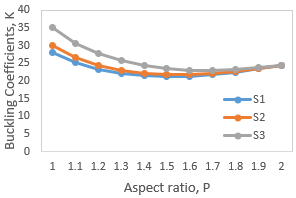Figure 4. CSCS stiffened panels, γ = 10, δ =0.1

## 5.png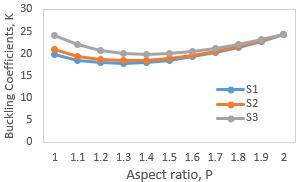Figure 5. CSCS stiffened panels, γ = 5, δ =0.05

## 6.png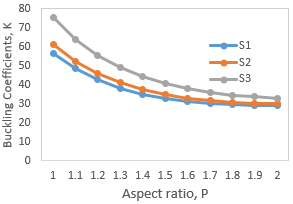Figure 6. CCCC stiffened panels, γ = 5, δ =0.05

## 7.png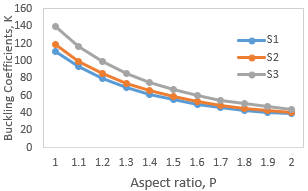Figure 7. CCCC stiffened panels, γ = 15, δ =0.2

## 8.png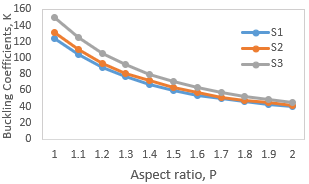Figure 8. CCCC stiffened panels, γ = 20, δ =0.3

Figures 7-8 show the result of CCCC stiffened at varying stiffness properties. It can be observed that the Numerical values presented for the CCCC and CSCS boundary conditions have similar sequence. The charts presented gives buckling behavior of isotropic stiffened panels under uni-axial loading and can be used to predict buckling resistivity of stiffeners with accuracy of exact deflection functions.

5. Conclusions

From the results of this study, the following conclusions can be drawn:

(I). The new method presented was used to provide analytical solutions for the elastic buckling analysis of rectangular stiffened panels subjected to uniform uniaxial in-plane compression for SSSS, CCCC, and CSCS boundary conditions. Exact deflection functions were directly substituted into buckling solutions derived to obtain buckling coefficients and the results shows high computational convergence.

(II). The stiffeners were equally spaced for the three boundary conditions considered. As expected CCCC boundary condition gives higher critical buckling coefficients. It implies that, buckling coefficients for stiffened panels increases when the plate is well supported.

(III). The main increase in the critical buckling coefficients occurs when the number of stiffeners increases and significant changes occurs in the buckling confidents when aspect ratio and stiffness parameters are increased.

(IV). The results obtained can serve as data comparison in applied research on the mechanics of continuum with stiffened panels and it can also provide designers with solutions for complex boundary conditions. Hence, the proposed method could be extended to elastic buckling of rectangular stiffened panels of free edges boundary conditions under uniform in-plane compressive loading.

Nomenclature
 a, b length and width of the plate C clamped edge CCCC all edges clamped CSCS two opposite edges clamped, two opposite edge simply supported D flexural rigidity E Young’s modulus h shape function K buckling coefficient P aspect ratio R, Q non dimensional coordinates along the x and y directions S simply supported edge SSSS all edges simply supported w deflection function x,y Cartesian coordinates in the horizontal and vertical direction, respectively dx, dy derivative in x and y directions Ai cross-sectional area of the stiffeners $\Pi_{\mathrm{P}}$ total potential energy functional for plates $\Pi_{s}$ total potential energy functional for stiffener $N_{x}$ compressive loads $\gamma_{i}$ ratio of bending stiffness rigidity of stiffeners $\delta_{i}$ ratio of cross-sectional area of the stiffeners to the plate Ii moment of inertia of stiffeners $\eta i$ distance of the stiffeners from the edge y = 0 Subscripts cri critical i number of points on the continuum
References

 Bisagni, C., Vescovini, R. (2009). Analytical formulation for local buckling and post-buckling analysis of stiffened laminated panels. Thin-Walled Structures, 47(3): 318-334. https://doi.org/10.1016/j.tws.2008.07.006

 Bedair, O. (2009). Analysis and limit state design of stiffened plates and shells: A world view. Applied Mechanics Reviews, 62(2): 1-16. https://doi.org/10.1115/1.3077137

 Galéa, Y., Martin, P.O. (2010). Longitudinally stiffened plates in Eurocode 3: Calculation of the global critical buckling stress. Journal of Constructional Steel Research, 66(11): 1345-1353. https://doi.org/10.1016/j.jcsr.2010.05.001

 Rahbar-Ranji, A. (2013). Elastic buckling analysis of longitudinally stiffened plates with flat-bar stiffeners. Ocean Engineering, 58: 48-59. https://doi.org/10.1016/j.oceaneng.2012.09.018

 Brubak, L., Hellesland, J. (2013). Thin-walled structures ultimate strength prediction by semi-analytical analysis of stiffened plates with various boundary conditions. Thin-Walled Structures, 62: 28-36. https://doi.org/10.1016/j.tws.2012.08.005

 Ibearugbulem, M.O., Ibeabuchi, V.T., Njoku, K. (2014). Buckling analysis of SSSS stiffened rectangular isotropic plates using work principle approach. International Journal of Innovative Research and Development, 3(11): 169-176.

 Oliveri, V., Milazzo, A. (2018). A Rayleigh-Ritz approach for postbuckling analysis of variable angle tow composite stiffened panels. Computers and Structures, 196: 263-276. https://doi.org/10.1016/j.compstruc.2017.10.009

 Milazzo, A., Benedetti, I., Gulizzi, V. (2018). An extended Ritz formulation for buckling and post-buckling analysis of cracked multilayered plates. Composite Structures, 201: 980-994. https://doi.org/10.1016/j.compstruct.2018.06.026

 Guo, Y.T., Nie, X., Tao, M.X., Ding, R., Tang, L., Fan, J.S. (2019). Selected series method on buckling design of stiffened steel-concrete composite plates. Journal of Constructional Steel Research, 161: 296-308. https://doi.org/10.1016/j.jcsr.2019.07.014

 Deng, J., Wang, X., Yuan, Z., Zhou, G. (2019). Novel quadrature element formulation for simultaneous local and global buckling analysis of eccentrically stiffened plates. Aerospace Science and Technology, 87: 154-166. https://doi.org/10.1016/j.ast.2019.02.019

 Li, S., Hu, Z., Benson, S. (2019). An analytical method to predict the buckling and collapse behaviour of plates and stiffened panels under cyclic loading. Engineering Structures, 199: 109627. https://doi.org/10.1016/j.engstruct.2019.109627

 Zhou, W., Li, Y., Shi, Z., Lin, J. (2019). An analytical solution for elastic buckling analysis of stiffened panel subjected to pure bending. International Journal of Mechanical Sciences, 161-162: 105024. https://doi.org/10.1016/j.ijmecsci.2019.105024

 Ibeabuchi, V.T., Ibearugbulem, O.M., Ezeah, C., Ugwu, O.O. (2020). Elastic buckling analysis of uniaxially compressed CCCC stiffened isotropic plates. International Journal of Applied Mechanics and Engineering, 25(4): 84-95. https://doi.org/10.2478/ijame-2020-0051

 Timoshenko, S.P., Gere, J.M. (1961). Theory of Elastic Stability, Second Edition. In Journal of Applied Mechanics. McGraw-Hill. https://doi.org/10.1115/1.3636481

 Ventsel, E., Krauthammer, T. (2001). Thin Plates and Shells  Theory, Analysis, and Applications. Marcel Dekker, New York. https://doi.org/10.1201/9780203908723

 Wang, C.M., Aung, T.M. (2007). Plastic buckling analysis of thick plates using p-Ritz method. International Journal of Solids and Structures, 44: 6239-6255. https://doi.org/10.1016/j.ijsolstr.2007.02.026

 Ibearugulem Owus, M. (2011). Buckling analysis of axially compressed SSSS thin rectangular plate using Taylor-Mclaurin shape function. International Journal of Civil and Structural Engineering, 2(2): 676-681. https://doi.org/10.6088/ijcser.00202010142

 Iyengar, N.G. (1988). Structural Stability of Columns and Plates. Ellis Horwood, New York, pp. 179-185.